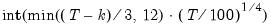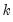coint
Test for cointegration between series in an equation.
Test for cointegration between series in an equation estimated by Equation::cointreg. You may perform a Hansen Instability Test, Park Added Variable (Spurious Trends) Test, or between a residual-based Engle-Granger or Phillips-Ouliaris test.
Johansen tests for cointegration may be performed from a group or a VAR object (see Group::coint and Var::coint).
The cointegrating equation specification is taken from the equation. Additional test specification components are specified as options and arguments.
Syntax
Equation View: eq_name.coint(options) [arg]
where

 method=arg (default=“hansen”) Test method: Hansen’s Instability test (“hansen”), Park’s Added Variable (“park”), Engle-Granger residual test (“eg”), Phillips-Ouliaris residual test (“po”).
and arg is an optional list describing additional regressors to include in the Park Added Regressors test (when “method=park” is specified).
The Park, Engle-Granger, and Phillips-Ouliaris tests all have options which control various aspects of the test.
Options
Options for the Park Test
The following option, along with the optional argument described above, determines the additional regressors to include in the test equation.

 trend=arg (default=two orders higher than trend in estimated equation) Specification for the powers of trend to include in the test equation: None (“none”), Constant (“const”), Linear trend (“linear”), Quadratic trend (“quadratic”), Cubic trend (“cubic”), Quartic trend (“quartic”), integer (user-specified power).Note that the specification implies all trends up to the specified order so that choosing a quadratic trend instructs EViews to include a constant and a linear trend term along with the quadratic. Only trend orders higher than those specified in the original equation will be considered. p Print results.
Options for the Engle-Granger Test
The following options determine the specification of the Engle-Granger test (Augmented Dickey-Fuller) equation and the calculation of the variances used in the test statistic.

 lag=arg (default=“a”) Method of selecting the lag length (number of first difference terms) to be included in the regression: “a” (automatic information criterion based selection), or integer (user-specified lag length). lagtype=arg (default=“sic”) Information criterion or method to use when computing automatic lag length selection: “aic” (Akaike), “sic” (Schwarz), “hqc” (Hannan-Quinn), “msaic” (Modified Akaike), “msic” (Modified Schwarz), “mhqc” (Modified Hannan-Quinn), “tstat” (t-statistic). maxlag=integer Maximum lag length to consider when performing automatic lag-length selectiondefault=whereis the number of coefficients in the cointegrating equation. Applicable when “lag=a”. lagpval=number (default=0.10) Probability threshold to use when performing automatic lag-length selection using a t-test criterion. Applicable when both “lag=a” and “lagtype=tstat”. nodf Do not degree-of-freedom correct estimates of the variances. p Print results.
Options for the Phillips-Ouliaris Test
The following options control the computation of the symmetric and one-sided long-run variances in the Phillips-Ouliaris test.
Basic Options

 nodf Do not degree-of-freedom correct the coefficient covariance estimate. p Print results.
HAC Whitening Options

 lag=arg (default=0) Lag specification: integer (user-specified lag value), “a” (automatic selection). infosel=arg (default=“aic”) Information criterion for automatic selection: “aic” (Akaike), “sic” (Schwarz), “hqc” (Hannan-Quinn) (if “lag=a”). maxlag=integer Maximum lag-length for automatic selection (optional) (if “lag=a”). The default is an observation-based maximum.
HAC Kernel Options

 kern=arg (default=“bart”) Kernel shape: “none” (no kernel), “bart” (Bartlett, default), “bohman” (Bohman), “daniell” (Daniel), “parzen” (Parzen), “parzriesz” (Parzen-Riesz), “parzgeo” (Parzen-Geometric), “parzcauchy” (Parzen-Cauchy), “quadspec” (Quadratic Spectral), “trunc” (Truncated), “thamm” (Tukey-Hamming), “thann” (Tukey-Hanning), “tparz” (Tukey-Parzen). bw=arg (default=“nwfixed”) Bandwidth: “fixednw” (Newey-West fixed), “andrews” (Andrews automatic), “neweywest” (Newey-West automatic), number (User-specified bandwidth). nwlag=integer Newey-West lag-selection parameter for use in nonparametric bandwidth selection (if “bw=neweywest”). bwoffset=integer (default=0) Apply integer offset to bandwidth chosen by automatic selection method (“bw=andrews” or “bw=neweywest”). bwint Use integer portion of bandwidth chosen by automatic selection method (“bw=andrews” or “bw=neweywest”).
Examples
Hansen
base_eq.coint
estimates the cointegrating equation BASE_EQ using FMOLS and performs the Hansen cointegration test.
Park
base_eq.coint(method=park)
conducts the default Park test, which for BASE_EQ involves testing the significance of the quadratic and cubic trend coefficients.
base_eq.coint(method=park, trend=quartic) mytrend
performs a test which evaluates the significance of the quadratic, cubic, and quartic terms, and user trend variable MYTREND.
base_eq.coint(method=eg, trend=6)
estimates the test equation with trend powers up to 6.
Engle-Granger
base_eq.coint(method=eg)
performs the default Engle-Granger test using SIC and an observation-based maximum number of lags to determine the lags for an ADF equation.
base_eq.coint(method=eg, lag=a, lagtype=tstat, lagpval=.15, maxlag=10)
uses a sequential t-test starting at lag 10 with threshold probability 0.15 to determine the number of lags.
base_eq.coint(method=eg, lag=5)
conducts an Engle-Granger cointegration test with a fixed lag of 5.
Phillips-Ouliaris
base_eq.coint(method=po)
performs the default Phillips-Ouliaris test using a Bartlett kernel and Newey-West fixed bandwidth.